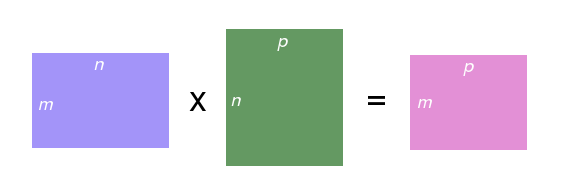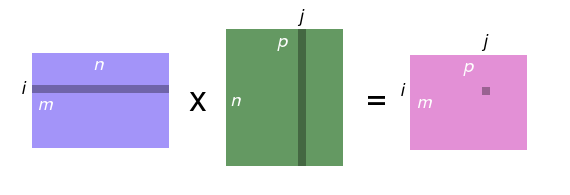Storage 4: Processor caches and forking

Overview

In this lecture, we work through some examples of processor cache performance and transition to the process control unit.

Processor cache

• Processor caches divide primary memory into blocks of ~64 aligned bytes
• 128 bytes on some machines
• Processor implements prefetching strategies

accessor.cc

• Initialize array of N integers
• Sum up N elements of the array in one of three orders
• -u (up order): 0…(N-1)
• -d (down order): (N-1)…0
• -r (random order): N random choices from [0, N-1)

Question

• What is the relative speed of these orders, and why?

list-inserter.cc

• Initialize sorted linked list of N integers
• Insert N elements in one of three orders
• -u (up order): 0…N-1
• -d (down order): N-1…0
• -r (random order): N random choices from [0, N-1)

Question

• What is the relative speed of these orders, and why?

Machine learning Matrix multiplication

• Matrix is 2D array of numbers
• m\times n matrix M has m rows and n columns
• M_{ij} is the value in row i and column j
• Product of two matrices C = A \times B
• If A is m\times n, then B must have dimensions n\times p (same number of rows as A has columns), and C has dimensions m \times p
• C_{ij} = \sum_{0\leq k < n} A_{ik}B_{kj}

Pictorial matrix multiplicationMatrix storage

• How to store a 2D matrix in “1D” memory?
• Row-major order
• Store row values contiguously in memory
• Single-array representation
• MxN matrix stored in array m[M*N]
• Matrix element M_{ij} stored in array element m[i*N + j]
• 4x4 matrix: A_{0,0} := a; A_{0,1} := a; A_{0,2} := a; …; A_{3,2} := a; A_{3,3} := a

Implementing matrix multiplication

// clear c
for (size_t i = 0; i != c.size(); ++i) {
for (size_t j = 0; j != c.size(); ++j) {
c.at(i, j) = 0;
}
}

// compute product and update c
for (size_t i = 0; i != c.size(); ++i) {
for (size_t j = 0; j != c.size(); ++j) {
for (size_t k = 0; k != c.size(); ++k) {
c.at(i, j) += a.at(i, k) * b.at(k, j);
}
}
}

Question

• Can you speed up matrixmultiply.cc by 10x?